Read- Identify Arithmetic Patterns Video for Kids | 3th, 4th & 5th Grade
1%
It was processed successfully!

# Read About Identifying Number Patterns (In Arithmetic and the Multiplication Table)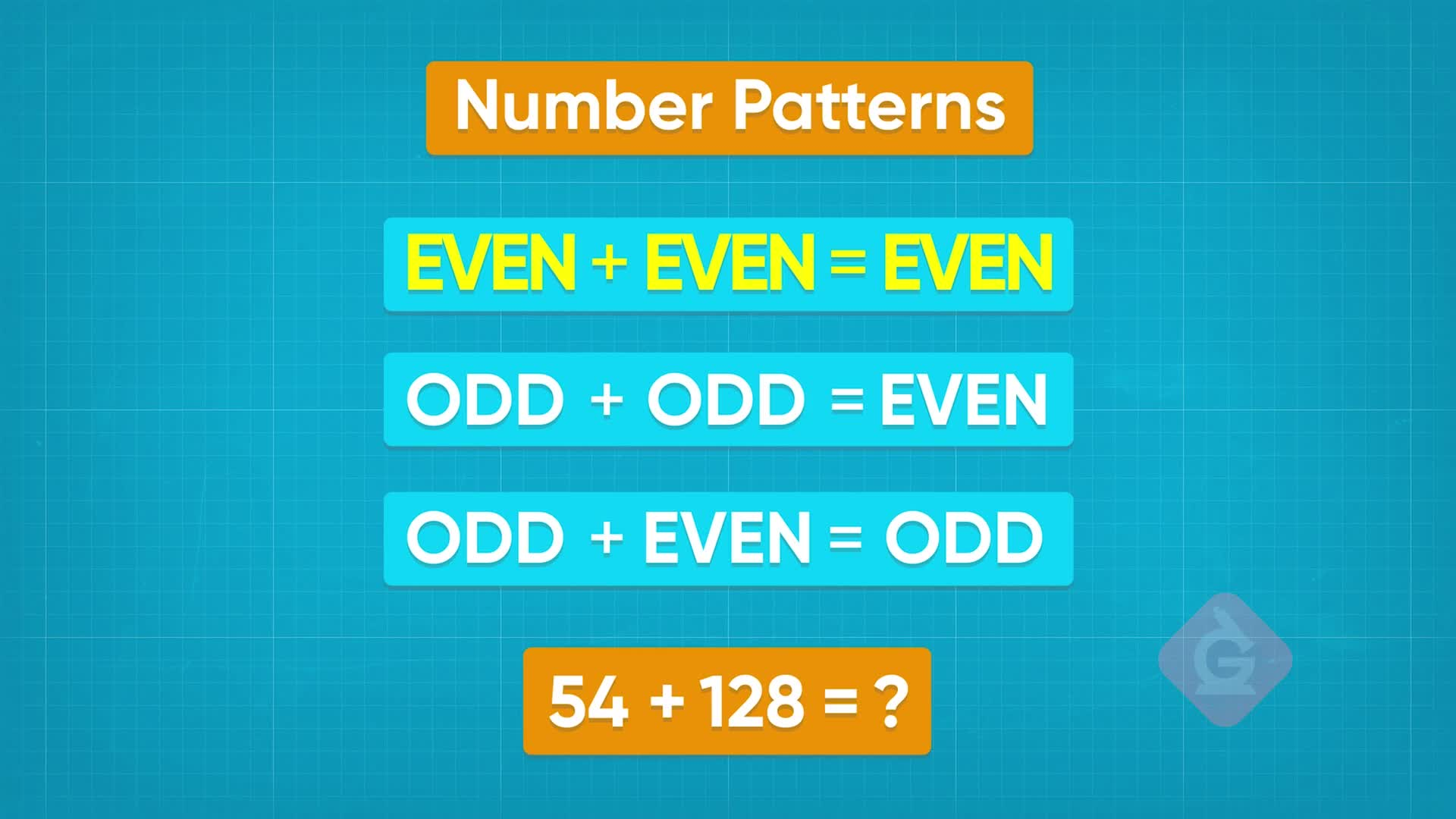WHAT IS IDENTIFYING NUMBER PATTERNS (IN ARITHMETIC AND THE MULTIPLICATION TABLE)

Having explored patterns with shapes, you will now discover that patterns can be found in numbers as well! Specifically, you will explore outcomes when even and odd numbers are added, subtracted, and multiplied.

To better understand identifying number patterns…

WHAT IS IDENTIFYING NUMBER PATTERNS (IN ARITHMETIC AND THE MULTIPLICATION TABLE). Having explored patterns with shapes, you will now discover that patterns can be found in numbers as well! Specifically, you will explore outcomes when even and odd numbers are added, subtracted, and multiplied. To better understand identifying number patterns…

## LET’S BREAK IT DOWN!

### Odd and Even Socks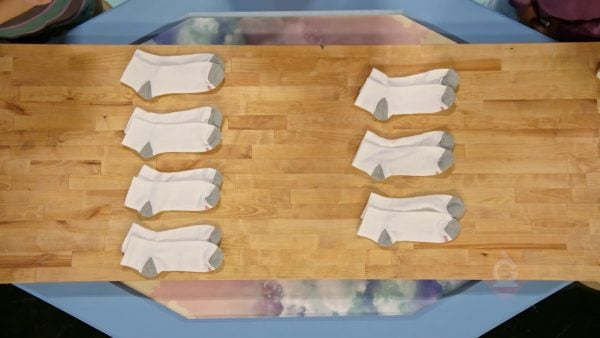Adesina, April, and Marcos are folding laundry. Each person has several socks, and they want to know how they can tell if it’s an even or odd number. If we can put all the socks into pairs, then there is an even number. April has 6 socks, and they can be put into 3 pairs, so 6 is even. Marcos has 8 socks, and they can all be paired up as well, so 8 is even. Combined, they have 14 pairs of socks, and 14 socks can all be paired up as well, so that number is even. They have just discovered a pattern: Even + even = even, since there are no left overs. Next, they fold socks that are a different color. This time, Marcos has 5 socks and April has 9 socks. 5 is an odd number because one of the socks is not paired. 9 is also an odd number because one of the socks is unpaired. But, if Marcos and Adesina put their socks together, now their unpaired socks make a pair together! Therefore, odd + odd = even. Next, they work on a new pile of socks. This time, Marcos has 6 socks and April has 5 socks. 6 is even, since Marcos' socks can all be paired. 5 is odd, since April has one sock left unpaired. If they combine their socks, one sock is still unpaired, and the answer is still odd. Therefore, odd + even = odd! Now you try: Without calculating, can you tell if 7 + 12 is odd or even?

Odd and Even Socks Adesina, April, and Marcos are folding laundry. Each person has several socks, and they want to know how they can tell if it’s an even or odd number. If we can put all the socks into pairs, then there is an even number. April has 6 socks, and they can be put into 3 pairs, so 6 is even. Marcos has 8 socks, and they can all be paired up as well, so 8 is even. Combined, they have 14 pairs of socks, and 14 socks can all be paired up as well, so that number is even. They have just discovered a pattern: Even + even = even, since there are no left overs. Next, they fold socks that are a different color. This time, Marcos has 5 socks and April has 9 socks. 5 is an odd number because one of the socks is not paired. 9 is also an odd number because one of the socks is unpaired. But, if Marcos and Adesina put their socks together, now their unpaired socks make a pair together! Therefore, odd + odd = even. Next, they work on a new pile of socks. This time, Marcos has 6 socks and April has 5 socks. 6 is even, since Marcos' socks can all be paired. 5 is odd, since April has one sock left unpaired. If they combine their socks, one sock is still unpaired, and the answer is still odd. Therefore, odd + even = odd! Now you try: Without calculating, can you tell if 7 + 12 is odd or even?

### Googly Eyes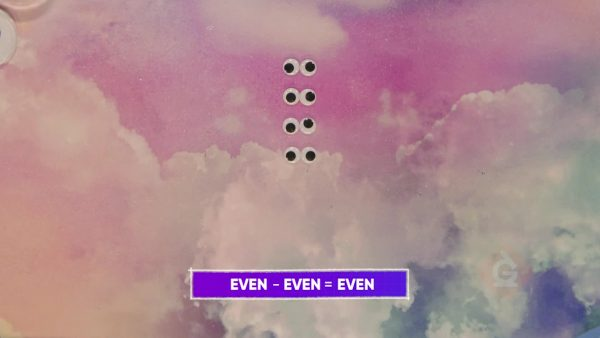Adesina starts by putting 12 googly eyes into pairs. 12 can be put into 6 pairs, so 12 is even. If we subtract 4, which is an even number, then we have 8 googly eyes left, which is also even. Subtracting an even number from an even number doesn't leave any googly eyes unpaired. Even – even = even. If we try subtracting an odd number from an odd number, like 11 – 5 = 6, we end up with an even number! The unpaired googly eye in 11 is taken away by the unpaired eye in 5. Odd – odd = even. If we subtract an odd number from an even number, like 14 – 3 = 11, we get an odd number. Likewise, if we subtract an even number from an odd number, like 13 – 4 = 9, we also get an odd number. Then, even – odd = odd, and odd – even = odd. Now you try: Without calculating, can you tell if 21 – 9 is even or odd?

Googly Eyes Adesina starts by putting 12 googly eyes into pairs. 12 can be put into 6 pairs, so 12 is even. If we subtract 4, which is an even number, then we have 8 googly eyes left, which is also even. Subtracting an even number from an even number doesn't leave any googly eyes unpaired. Even – even = even. If we try subtracting an odd number from an odd number, like 11 – 5 = 6, we end up with an even number! The unpaired googly eye in 11 is taken away by the unpaired eye in 5. Odd – odd = even. If we subtract an odd number from an even number, like 14 – 3 = 11, we get an odd number. Likewise, if we subtract an even number from an odd number, like 13 – 4 = 9, we also get an odd number. Then, even – odd = odd, and odd – even = odd. Now you try: Without calculating, can you tell if 21 – 9 is even or odd?

### Multiplication Table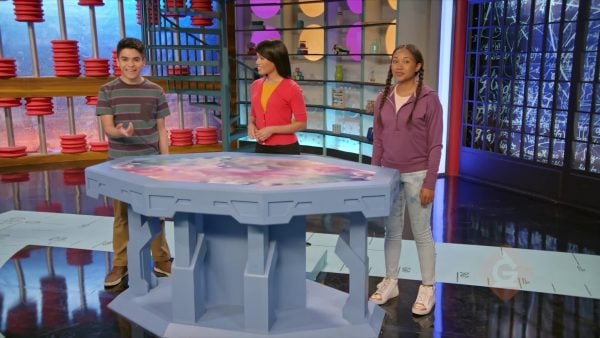Adesina, Marcos, and April are looking at a multiplication table. They notice some interesting number patterns: The row showing multiples of 2 contains all even numbers. All multiples of 2 are even! Looking at the row with multiples of 5, they notice that you add 5 each time you move along the row. All multiples of 5 end in 5 or 0. This is how you know you cannot divide 23 by 5: it doesn’t end in 5 or 0. Looking at the row with multiples of 10, we can see that all multiples of 10 end in 0. Discovering these patterns can help us check our work. Now you try: Is 73 divisible by 5? Is 90 divisible by 10? Is 43 divisible by 2?

Multiplication Table Adesina, Marcos, and April are looking at a multiplication table. They notice some interesting number patterns: The row showing multiples of 2 contains all even numbers. All multiples of 2 are even! Looking at the row with multiples of 5, they notice that you add 5 each time you move along the row. All multiples of 5 end in 5 or 0. This is how you know you cannot divide 23 by 5: it doesn’t end in 5 or 0. Looking at the row with multiples of 10, we can see that all multiples of 10 end in 0. Discovering these patterns can help us check our work. Now you try: Is 73 divisible by 5? Is 90 divisible by 10? Is 43 divisible by 2?

### Multiplying Evens and Odds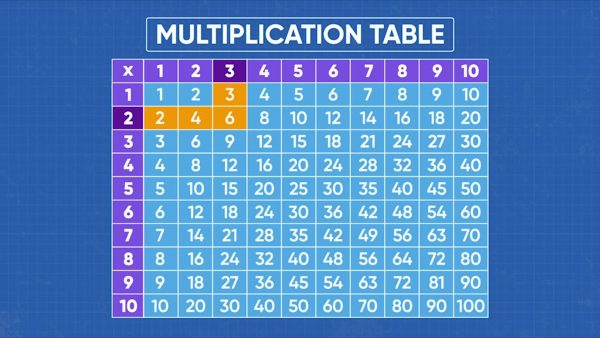Let’s see what happens when we multiply using even and odd numbers. To find the product of two numbers, we can look up the two numbers on the multiplication table to see what they multiply to. 2 and 4 are even, and when we multiply them, we get 8, which is also an even number. Then, even × even = even. 1 and 3 are odd, and if we multiply them, we get 3, an odd number. Same with 5 × 7 = 35. Then, odd × odd = odd. 2 is even and 3 is odd, and if we multiply them, we get 6, an even number. Same with 6 × 9 = 54. Then, even × odd = even. Also, odd × even = even. Now you try: Without calculating, can you tell if 11 × 16 is odd or even?

Multiplying Evens and Odds Let’s see what happens when we multiply using even and odd numbers. To find the product of two numbers, we can look up the two numbers on the multiplication table to see what they multiply to. 2 and 4 are even, and when we multiply them, we get 8, which is also an even number. Then, even × even = even. 1 and 3 are odd, and if we multiply them, we get 3, an odd number. Same with 5 × 7 = 35. Then, odd × odd = odd. 2 is even and 3 is odd, and if we multiply them, we get 6, an even number. Same with 6 × 9 = 54. Then, even × odd = even. Also, odd × even = even. Now you try: Without calculating, can you tell if 11 × 16 is odd or even?

## IDENTIFY NUMBER PATTERNS (IN ARITHMETIC AND THE MULTIPLICATION TABLE) VOCABULARY

Pattern
Something that follows a rule.
Combined
Pair
Two of the same thing.
Sequence
When one thing follows another according to a rule.
Equation
Mathematical expressions that are equal to the same number.
Multiplication table
A tool that helps us quickly see the product of two numbers.
Can be put into two equal groups, or into groups of two.
Cannot be put into two equal groups, or into groups of two.

## IDENTIFY NUMBER PATTERNS (IN ARITHMETIC AND THE MULTIPLICATION TABLE) DISCUSSION QUESTIONS

### If I have 4 socks and you have 8 socks, can we pair them all up if we put them together?

Yes! 4 can be put into pairs, and 8 can be put into pairs, so if we put them together, we are just adding pairs.

### If I have 5 socks and you have 11 socks, can we pair them all up if we put them together?

Yes. All my socks can be put into pairs except one. All your socks can be put into pairs except one. Together, we have several pairs of socks and two unpaired socks. Those unpaired socks become a pair together!

### If you start with 12 socks and take away 3 socks, you have 9 socks left. Which rule can you learn from this story?

I started with an even number of socks that can be put into pairs. Then I took away an odd number of socks, which means that I take away some pairs and also break up a pair to take one sock. That means that I have an unpaired sock left over, so the amount of leftover socks is an odd number. The rule is: even – odd = odd.

### What happens if you multiply an even number by an odd number? Explain.

I get an even number! I can think of it as an odd number of groups of even numbers (which can be paired).

### What happens if you multiply an odd number by an odd number? Explain.

I get an odd number. For example, if I have 3 groups that each contain 5 socks, the socks in each group make 2 pairs with 1 sock unpaired. When I combine the groups, there are 3 unpaired socks, which still leaves 1 unpaired.
X

## Success

We’ve sent you an email with instructions how to reset your password.
Ok

## Choose Your Free Trial Period### 7 DaysContinue to Lessons### 90 DaysGet 90 days free by inviting other teachers to try it too.

Share with Teachers

## Get 90 Days Free

### By inviting 4 other teachers to try it too.

4 required

Skip, I will use a 7 days free trial

## Thank You!

Enjoy your free 90 days trial